# TensorFlow2.1入门学习笔记(1)——Numpy科学计算库

### 1.多为数组的形状(Shape)

#### 一维数组 shape:(5,)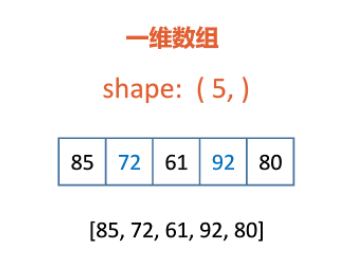#### 二维数组 shape:(30,5)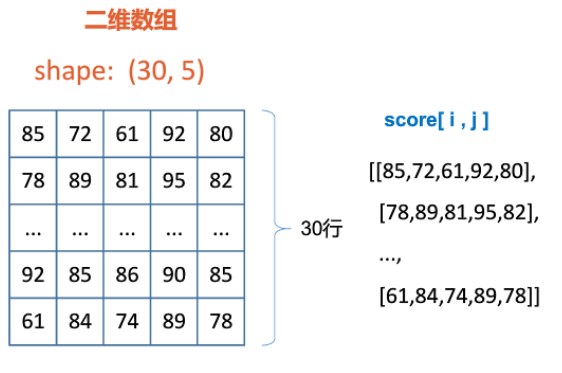#### 三维数组 shape:(10,30,5)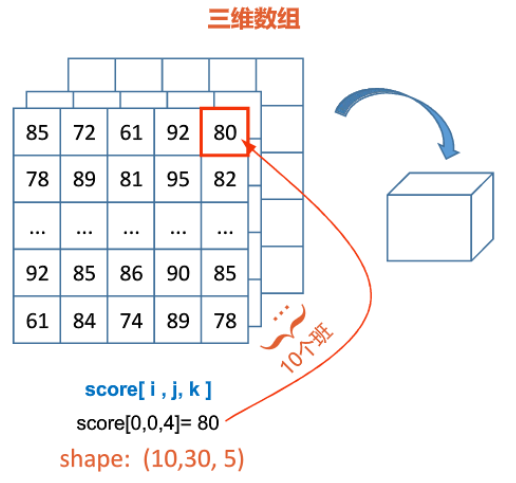#### 四维数组 shape:(5,10,30,5)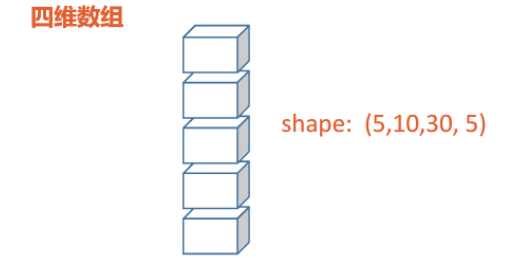#### 五维数组 shape:(4,5,10,30,5)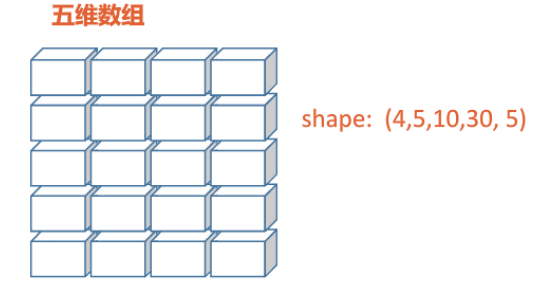更高维以此类推

### 2.创建Nump

#### 安装Numpy库

 1  pip install -i https://pypi.tuna.tsinghua.edu.cn/simple numpy 

#### 导入Numpy库

 1 2  import numpy as np import numpy import * #可直接调用库，但不推荐，容易和其他包冲突 

#### 创建数组

 1 2 3 4 5 6 7 8  m = np.array([[[4, 5, 8, 3],[3, 6, 9, 0],[8, 4, 5, 6]], [[4, 5, 8, 3],[3, 6, 9, 0],[8, 4, 2, 1]]]) # 数组属性 m.ndim #3 维度 m.shape #(2,3,4) 形状 m.size #24 元素的总个数 m.dtype #int32 数据类型 m.itemsize #4 每个元素的字节数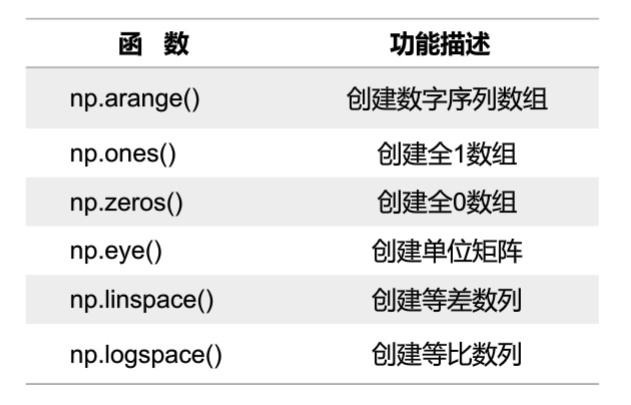1 2 3 4 5 6 7  # np.arrange(start=0,stop,num=1,dtype) 前闭后开，不包含结束值 n=np.arange(4) #array([0, 1, 2, 3]) a=np.arange(0,2,0.3) #array([0., 0.3, 0.6, 0.9, 1.2, 1.5, 1.8]) np.ones((3,2),dtype =np.int64) #array([[1,1],[1, 1],[1, 1]],dtype = int64) np.eye(2, 3) #array([[1., 0., 0.], [0., 1., 0.]])创建一个单位矩阵 # np.logspace(stat,stop,num,base,dtype)参数：起始指数，结束指数，基，元素数据类型，包含结束值 np.logspace(1, 5, 5, base=2) #array([2., 4., 8, 16, 32])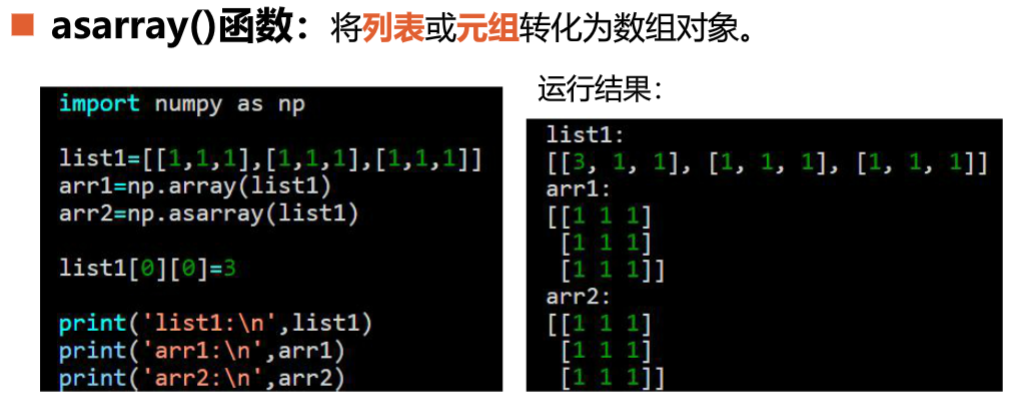### 3.数组计算

  1 2 3 4 5 6 7 8 9 10 11 12 13  # 数组元素切片 a = np.array([0,1,2,3]) #一维数组 print(a[:3]) #array([0,1,2]) 输出前三个数 b = np.array([[0,1,2,3],[3,4,5,6],[6,7,8,9]]) #二维数组 print(b[:2]) #array([[0,1,2,3],[3,4,5,6]]) 输出前两行 # 改变数组的形状 c = np.arange(12) d = c.reshape(3,4) print(d) #array([[0,1,2,3],[4,5,6,7],[8,9,10,11]]) 不改变当前数组，按照shape创建新的数组 c.reshape(-1,1) print(c) #array([,,,,,,,,,,,]) c.resize(3,4) print(c) #array([[0,1,2,3],[4,5,6,7],[8,9,10,11]]) 改变当前数组，按照shape创建新的数组 

#### 数组间的运算

1.数组间的元素运算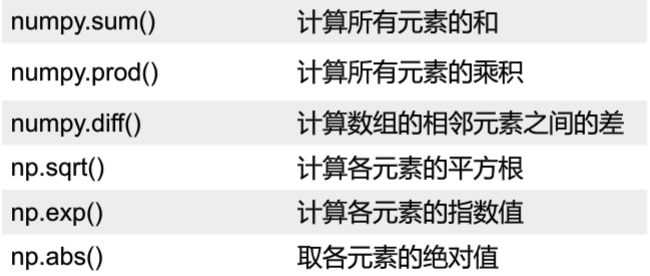1 2 3  a = np.arange(4) print(np.sum(a)) #6 print(np.sqrt(a)) #array([0. ,1. ,1.41421356, 1.73205081])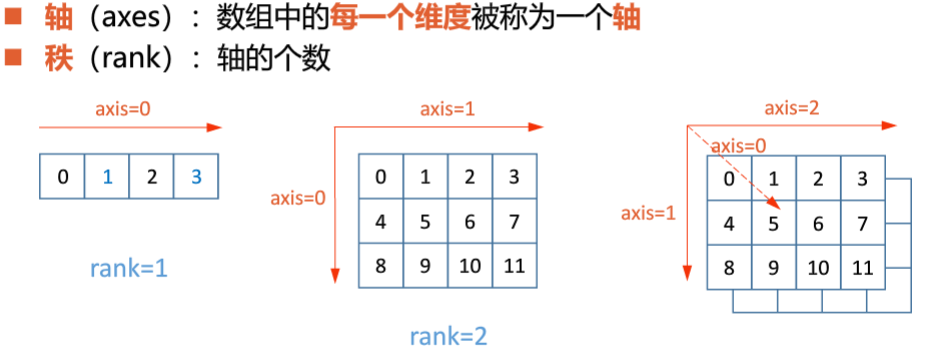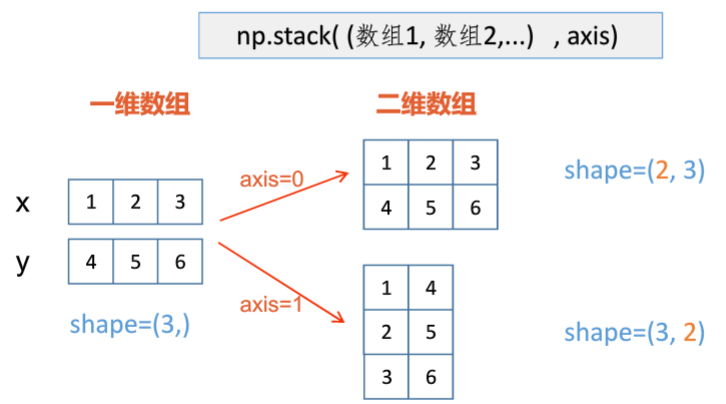1 2 3 4  x = np.array([1,2,3]) y = np.array([4,5,6]) print(np.stack((x,y),axis = 0)) #array([1,2,3],[4,5,6]) print(np.stack((x,y),axis = 1)) #array([1,4],[2,5],[3,6]) 
 1 2 3  a = np.arrange(12).reshape(3,4) #a = ([0,1,2,3],[4,5,6,7],[8,9,10,11]) print(np.sum(b,axis=0)) #array([12,15,18,21]) print(np.sum(b,axis=1)) #array([6,22,38]) 

2.数组加减法，对应元素相加减（进行运算的数组长度要一致）

 1 2 3  a = np.ones([3,3]) b = np.eye(3,3) print(a+b) #array([[2，1,1],[1,2,1],[1,1,2]]) 

3.一维数组可以和多维数组相加，相加时将会将一维数组扩展至多维

 1 2 3 4  a = np.array([1,2,3]) b = np.array([1,1,1],[2,2,2]) print(a+b) #array([2,3,4],[3,4,5]) print(b**2) #array([1,1,1],[4,4,4]) 

SUMMARIZE:数组间的四则运算，是对应元素加减乘除； 当数组中元素的数据类型不同时，精度低的数据类型会转换成精度高的数据类型，然后再运算

#### 矩阵运算

1.矩阵乘法，按矩阵相乘的规则运算

 1 2 3  A = np.array([[1,2],[2,3]]) B = np.array([[4,2,1],[1,5,2]]) print(np.matmul(A,B)) #array([6,12,5],[11,19,8]) 

2.转置和求逆

 1 2 3 4  #转置 print(np.transpose(A)) #array([1,2],[2,3]) #求逆 print(np.linalg.inv(A)) #array([-3,2],[2,-1])